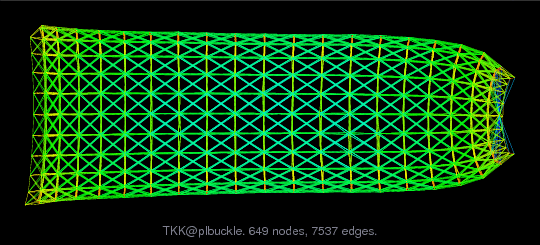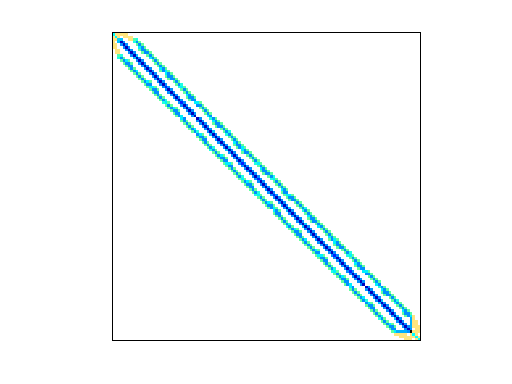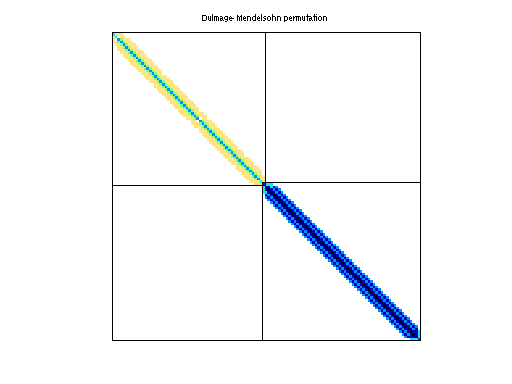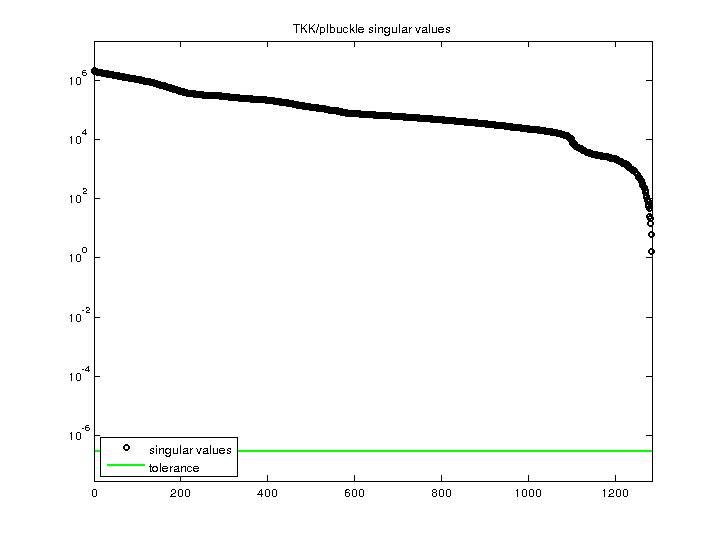Matrix: TKK/plbuckle

Description: Double eigenvalue plate buckling; stiffness matrix K and initial stress G(undirected graph drawing)• Matrix group: TKK
• download as a MATLAB mat-file, file size: 124 KB. Use UFget(1911) or UFget('TKK/plbuckle') in MATLAB.

 Matrix properties number of rows 1,282 number of columns 1,282 nonzeros 30,644 structural full rank? yes structural rank 1,282 # of blocks from dmperm 2 # strongly connected comp. 2 explicit zero entries 0 nonzero pattern symmetry symmetric numeric value symmetry symmetric type real structure symmetric Cholesky candidate? yes positive definite? yes

 author R. Kouhia editor T. Davis date 2008 kind structural problem 2D/3D problem? yes

 Additional fields size and type G sparse 1282-by-1282

Notes:

```Matrix problems from Reijo Kouhia, Structural Mechanics, Helsinki
University of Technology.   http://users.tkk.fi/~kouhia/sparse.html
```

 Ordering statistics: result nnz(chol(P*(A+A'+s*I)*P')) with AMD 43,827 Cholesky flop count 1.8e+06 nnz(L+U), no partial pivoting, with AMD 86,372 nnz(V) for QR, upper bound nnz(L) for LU, with COLAMD 44,989 nnz(R) for QR, upper bound nnz(U) for LU, with COLAMD 85,500

 SVD-based statistics: norm(A) 2.09715e+06 min(svd(A)) 1.63415 cond(A) 1.28332e+06 rank(A) 1,282 sprank(A)-rank(A) 0 null space dimension 0 full numerical rank? yes

 singular values (MAT file): click here SVD method used: s = svd (full (A)) ; status: ok